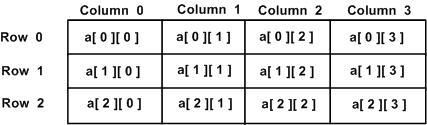# Multi-dimensional arrays in c

C programming language allows multidimensional arrays. Here is the general form of a multidimensional array declaration:

`type name[size1][size2]...[sizeN];`

For example, the following declaration creates a three dimensional 5 . 10 . 4 integer array:

`int threedim;`

## Two-Dimensional Arrays:

The simplest form of the multidimensional array is the two-dimensional array. A two-dimensional array is, in essence, a list of one-dimensional arrays. To declare a two-dimensional integer array of size x,y you would write something as follows:

`type arrayName [ x ][ y ];`

Where type can be any valid C data type and arrayName will be a valid C identifier. A two-dimensional array can be think as a table which will have x number of rows and y number of columns. A 2-dimensional array a, which contains three rows and four columns can be shown as below:Thus, every element in array a is identified by an element name of the form a[ i ][ j ], where a is the name of the array, and i and j are the subscripts that uniquely identify each element in a.

## Initializing Two-Dimensional Arrays:

Multidimensional arrays may be initialized by specifying bracketed values for each row. Following is an array with 3 rows and each row has 4 columns.

```int a = {
{0, 1, 2, 3} ,   /*  initializers for row indexed by 0 */
{4, 5, 6, 7} ,   /*  initializers for row indexed by 1 */
{8, 9, 10, 11}   /*  initializers for row indexed by 2 */
};```

The nested braces, which indicate the intended row, are optional. The following initialization is equivalent to previous example:

`int a = {0,1,2,3,4,5,6,7,8,9,10,11};`

## Accessing Two-Dimensional Array Elements:

An element in 2-dimensional array is accessed by using the subscripts, i.e., row index and column index of the array. For example:

`int val = a;`

The above statement will take 4th element from the 3rd row of the array. You can verify it in the above diagram. Let us check below program where we have used nested loop to handle a two dimensional array:

```#include <stdio.h>

int main ()
{
/* an array with 5 rows and 2 columns*/
int a = { {0,0}, {1,2}, {2,4}, {3,6},{4,8}};
int i, j;

/* output each array element's value */
for ( i = 0; i < 5; i++ )
{
for ( j = 0; j < 2; j++ )
{
printf("a[%d][%d] = %d\n", i,j, a[i][j] );
}
}
return 0;
}```

When the above code is compiled and executed, it produces the following result:

```a: 0
a: 0
a: 1
a: 2
a: 2
a: 4
a: 3
a: 6
a: 4
a: 8```

As explained above, you can have arrays with any number of dimensions, although it is likely that most of the arrays you create will be of one or two dimensions.